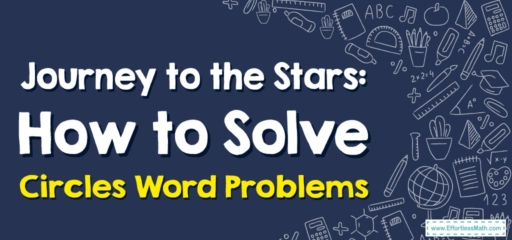# Journey to the Stars: How to Solve Circles Word Problems

Greetings, cosmic mathematicians!

Today, we're setting our course for the realm of circles. Circles are everywhere in our universe, from the shape of our planets to the orbits they trace around the sun. Now, let's embark on our interstellar journey!## 1. Interstellar Basics: Understanding Circles

Before we launch, let’s go over the celestial bodies of our journey:

• Circles: In the realm of math, a circle is a shape with all points the same distance from its center. Key aspects include the radius (distance from the center to the edge), diameter (distance across the circle through its center), and circumference (distance around the circle).

## 2. The Cosmic Journey: Word Problems about Circles

Now that we’re prepped for our journey, let’s launch into the world of circle word problems!

### Astronaut’s Guide: Navigating Word Problems about Circles

#### Step 1: Identify the Variables

First, we need to identify what the problem is asking us. Are we finding a radius, diameter, circumference, or maybe even the area?

#### Step 2: Use the Formulas

Next, we’ll use the appropriate formulas. Remember, the circumference of a circle is $$2πr$$ (or $$πd$$), and the area is $$πr²$$.

#### Step 3: Solve the Problem

Finally, it’s time to solve the problem. This may involve some algebra if we’re solving for a variable.

For instance, if the problem tells us the circumference of a planet’s orbit is $$6283\ km$$, what is the radius of this orbit?

1. Identify the Variables: We’re looking for the radius.
2. Use the Formulas: We know the formula for circumference is $$2πr$$.
3. Solve the Problem: Divide the circumference by $$2π$$ to find the radius. So, our radius is $$\frac{6283\ km}{2π} ≈ 1000\ km$$.

And that’s a successful journey through word problems about circles! Remember, mathematicians, our universe is filled with fascinating phenomena that can be understood better with math. Until our next cosmic journey, keep your eyes on the stars!

### What people say about "Journey to the Stars: How to Solve Circles Word Problems - Effortless Math: We Help Students Learn to LOVE Mathematics"?

No one replied yet.

X
30% OFF

Limited time only!

Save Over 30%

SAVE $5 It was$16.99 now it is \$11.99